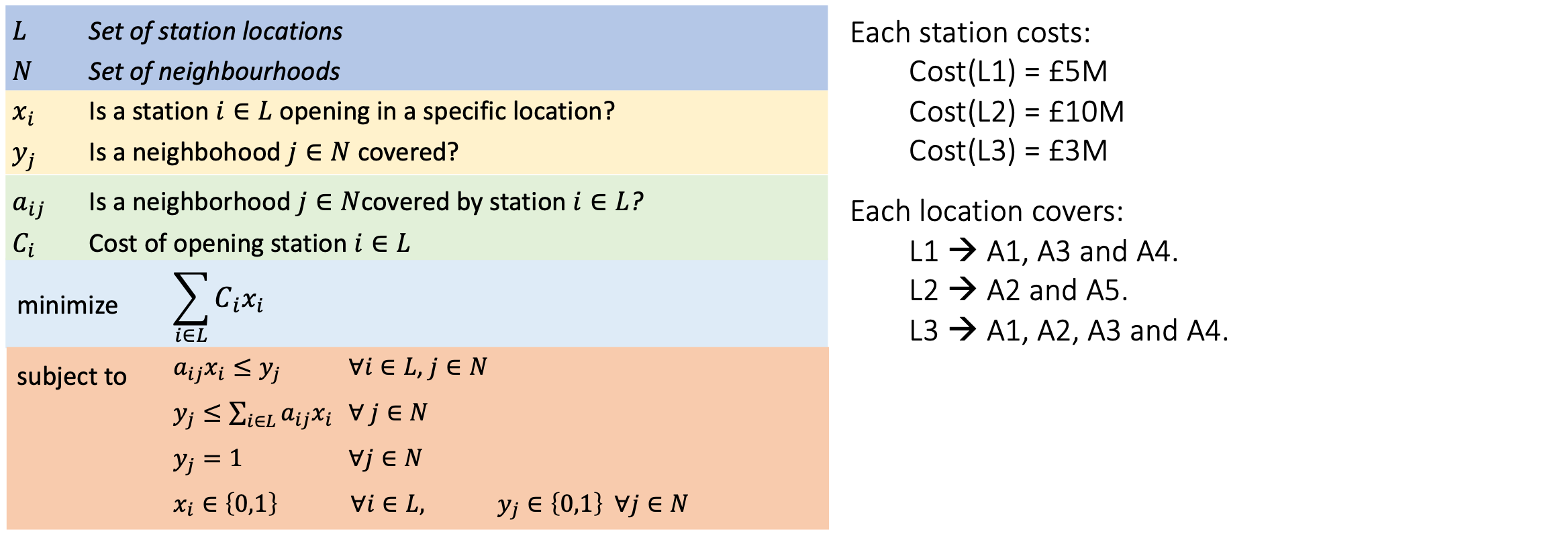# Notebook 3.8 - Covering Problem¶In :
# Setting up

from pulp import *

prob = LpProblem('prob', LpMinimize)

In :
L = ['L'+str(i+1) for i in range(3)]
N = ['A'+str(i+1) for i in range(5)]

In :
C = {'L1': 5, 'L2':10, 'L3':3}             # Cost dictionary

A = {'L1': {'A1':1, 'A3':1, 'A5':1},       # Coverage dictionary
'L2': {'A2':1, 'A5':1},
'L3': {'A1':1, 'A2':1, 'A3':1, 'A4':1}}


There are perhaps several way to define our coverage table - in our case, we chose to use a nested dictionary, with a value of 1 for the Station/Neighborhood pairs where coverage is available.

In :
# Declaring decision variables.

x = LpVariable.dicts('x', L, lowBound = 0, upBound = 1, cat = LpInteger)
y = LpVariable.dicts('y', N, lowBound = 0, upBound = 1, cat = LpInteger)


The decision variables in our problem are Boolean - we will therefore model them as integers, with a minimum value of 0, and a maximum value of 1.

In :
# Declaring objectives

prob += lpSum([C[i]*x[i] for i in L])

for i in L:
for j in N:
if j in A[i]:
prob += A[i][j]*x[i] <= y[j]

for j in N:
prob += y[j] <= lpSum(A[i][j]*x[i] for i in L if j in A[i] if i in A.keys() )

for j in N:
prob += y[j] == 1

In :
# Solving problem

prob.solve()

print('Total cost:', prob.objective.value())

print('')

print('Established Stations:\n')
for v in list(x.values()):
if v.varValue == 1:
print(f'  - {v.name.replace("x_","")} ')

Total cost: 8.0

Established Stations:

- L1
- L3


Everything in this notebook should be familiar to you, with the exception of the code that was used to iterate through the values in our list of decision variables.

In :
list(x.values())

Out:
[x_L1, x_L2, x_L3]

As we can see in the above cell, list(x.values()) can be used to obtain a list of our decision variable objects. This means that we can access each one directly using an iteration, or a direct call, such as this:

In :
list(x.values())

Out:
x_L1

To obtain the value that was obtained by our solver, we need to access the varValue property of our decision variable object:

In :
list(x.values()).varValue

Out:
1.0

## Summary¶

from pulp import *

prob = LpProblem('prob', LpMaximize)

S = [str(i+1) for i in range(4)]

revenues = [1000, 7000, 3000, 250]
weights  = [  50,  450,  100,  20]
volumes  = [ 0.5,    1,    2, 0.1]

R = dict(zip(S, revenues))
W = dict(zip(S, weights))
V = dict(zip(S, volumes))

W_max = 16200
V_max = 32

x = LpVariable.dicts('x', S,  lowBound = 0, cat = LpInteger)

prob += lpSum([R[i]*x[i] for i in S])

for i in S:
prob += lpSum([W[i]*x[i] for i in S]) <= W_max
prob += lpSum([V[i]*x[i] for i in S]) <= V_max

prob.solve()

for v in prob.variables():
print(f'  - Type {v.name.replace("x_","")} x {int(v.varValue):2}')

print('')

print('Total Revenue:', prob.objective.value())